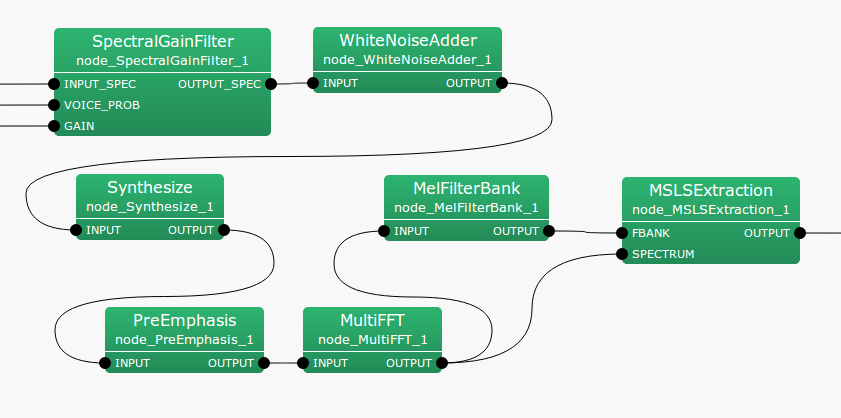### 6.7.42.1 Outline of the node

This node adds white noise to input signals.

### 6.7.42.2 Necessary files

No files are required.

### 6.7.42.3 Usage

When to use

This node is used to add white noise to input signals, thus reducing the influence of non-linear distortions after separation. For example, since PostFilter performs nonlinear processing, it is difficult to avoid musical noise, which may greatly influence the speech recognition performance. The influence of such noise can be reduced by adding an appropriate amount of known white noise.

Typical connection

An example is shown in the figure.Figure 6.154: Example of connection of WhiteNoiseAdder

### 6.7.42.4 Input-output and properties of the node

 Parameter name Type Default value Unit Description LENGTH 512 [pt] FFT length WN_LEVEL 0 Additional noise level

Input

INPUT

: Map<int, ObjectRef> type. Since ObjectRef is of Vector<complex<float> > type, signals of the frequency domain are input.

Output

OUTPUT

: Map<int, ObjectRef> type. Since ObjectRef is of Vector<complex<float> > type, signals to which white noise has been added are output.

Parameter

LENGTH

: FFT length; must be equal to the other nodes.

WN_LEVEL

: Additional noise level. Designate the maximum amplitude in the time domain.

### 6.7.42.5 Details of the node

The following is added to each frequency bin of input signals.

 \begin{equation} \frac{\sqrt {\rm LENGTH}}{2} \cdot {\rm WN\_ LEVEL} \cdot e^{2 \pi j R} \end{equation} (177)

$R$ indicates a random number of $0 \le R \le 1$ (different for each frequency bin). $\sqrt {\rm LENGTH}/2$ is used to revise distortions of scaling between the time and frequency domains that occur during frequency analysis by FFT.